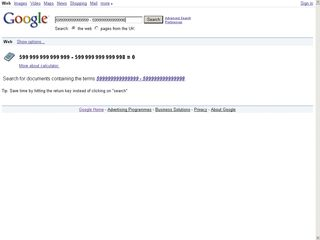Why computers suck at mathsComputers might struggle to exhibit intelligent behaviour, but blindly performing arithmetic calculations is surely their forte. Or is it?

The failure of Google's online calculator and Excel's apparent inability to give correct answers to simple calculations are both well-known problems among programmers, but these aren't really bugs in the normal sense of the word. Instead they're just a consequence of the fact that computers suck at maths.

Computers perform calculations in quite a different way from the methods that humans use to do arithmetic – and that means that they habitually come up with the wrong answer. Here we investigate some of the shocking consequences of this revelation before delving into the reason why computers suck at maths.

Close isn't close enough

For anyone still to be convinced that computers can't get simple arithmetic right, let's start off with a few examples that you can try out yourself.

First up, Google's calculator. If you've never tried it out before, to get a feel for how it works, surf to www.google.co.uk, type 5*9+(sqrt 9)^3 into the search box and click on 'Search'. You'll find that it comes back with the correct answer: '5 * 9 + (sqrt 9) ^3 = 72'.

Now let's try another calculation. Type in 599,999,999,999,999 - 599,999,999,999,998. Quite clearly, this should give an answer of 1. Unbelievably, however, Google responds with this: '599,999,999,999,999 – 599,999,999,999,998 = 0'. Just a rare and unfortunate example, perhaps?

OK then, let's try another simple calculation. Type =850*77.1 into cell A1 of an Excel 2007 workbook (it doesn't work – or should that be it does work – in earlier versions of Excel). A bit of mental arithmetic suggests that the answer ought to be in the region of 60,000; in fact the correct answer is 65,535.

Excel has other ideas. It will tell you that the result of this multiplication is 100,000, which is out by a massive 34,465. And to prove that this is no flash in the pan, how about using a selection of online calculators to work out 1.0 - 0.9 - 0.1?

You'll probably find at least half of them will come up with an answer of -2.77555756 E-17 – scientific notation for -0.0000000000000000277555756. (If all the ones you try give the right answer, take a look at www.calculator.net.)

BAD MATHS: Since 1.0 - 0.9 - 0.1 equals 0, why are so many online calculators convinced that this value is the answer instead?

OK, this answer might not be far removed from the correct answer of 0, but why can't the calculator come up with the right answer – an answer that's blatantly obvious to anyone who is conversant with simple arithmetic?

How computers do maths

Although computers can handle integers (whole numbers), for general-purpose arithmetic they store numbers in floating point format because it's so much more efficient in memory use.

Let's take the double precision floating point representation as an example. It uses 64 bits to store each number and permits values from about -10308 to 10308 (minus and plus 1 followed by 308 zeros, respectively) to be stored. Furthermore, fractional values as small as plus or minus 10-308 (that's a decimal point followed by 307 zeros and then a 1) can be stored.

By way of contrast, if the same 64 bits were used to store integers, the range would be −9,223,372,036,854,775,808 to +9,223,372,036,854,775,807, and fractional values couldn't be represented.

The secret to this apparently amazing efficiency is approximation. Of those 64 bits, one represents the sign (so whether the value is positive or negative), 52 bits represent the mantissa (that's the actual numbers) and the remaining 11 bits represent the exponent (how many zeros there are or where the decimal point is).

So although a much greater range of numbers can be stored using floating point notation, the precision is actually less than can be achieved in integer format, since only 52 bits are available. In fact, 52 bits of binary information represents a 16-bit decimal number, so any values that differ only in their 17th decimal point will actually be seen as identical.

The situation with Google thinking that 599,999,999,999,999 - 599,999,999,999,998 equals 0 is similar, although it's evident that Google's calculator actually uses less than the normal 52 bits for the mantissa. That some calculators give a non-zero result to the calculation 1.0 - 0.9 - 0.1 might seem different since we appear to be nowhere close to the limit of 64-bit floating point arithmetic.

But that's forgetting one important fact – that computers work in binary. And although 0.1 might have only one significant digit in decimal, in binary notation the mantissa is a repeating sequence. This means that 0.1 can never be represented accurately in binary, no matter how many bits you use.